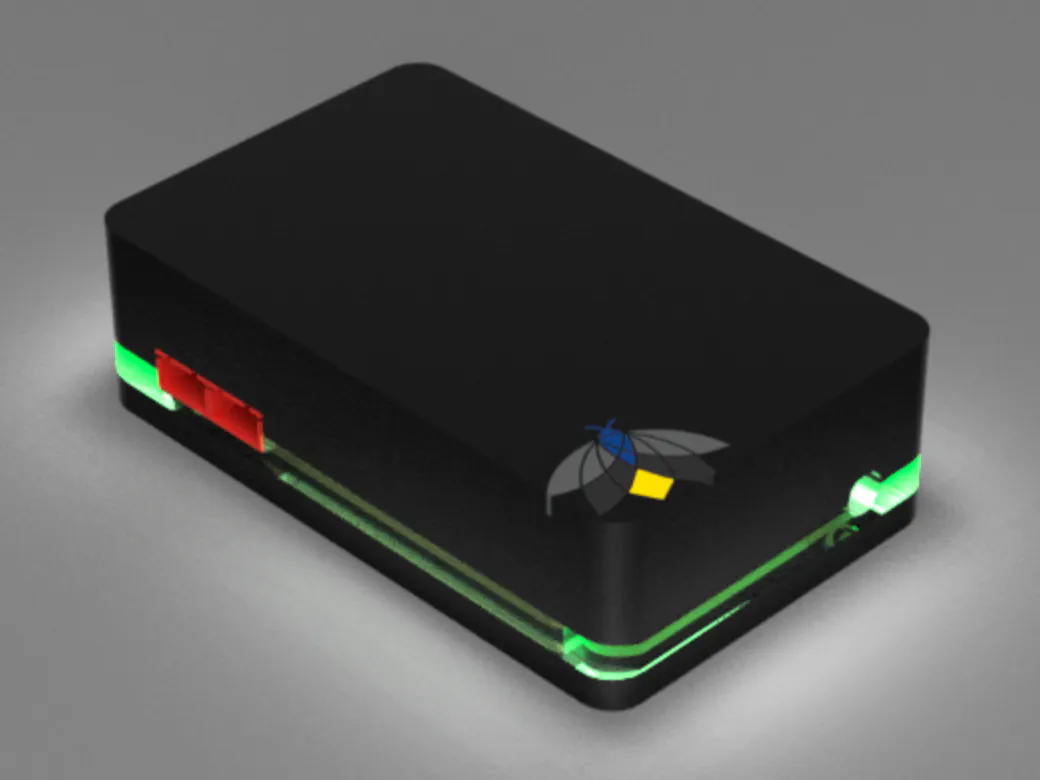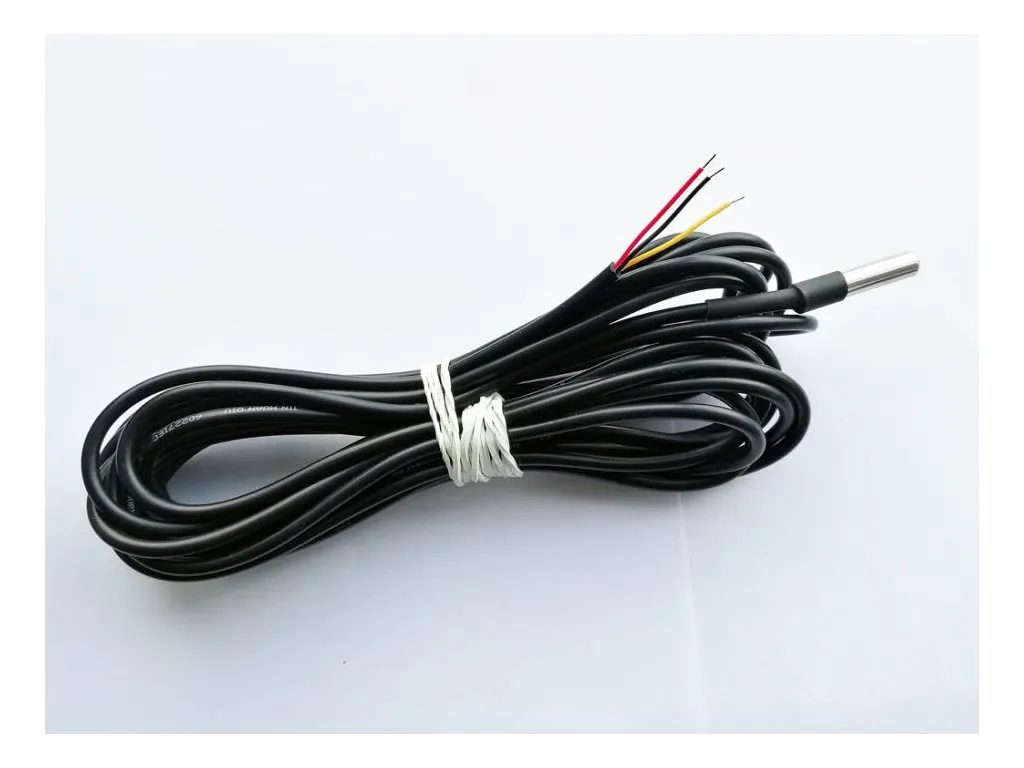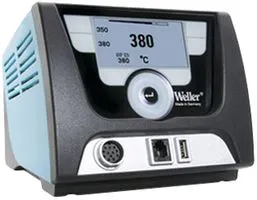# Pump Control and Monitor

Control any high voltage (240V, 15A) load whilst monitoring two external sensors and current drawn by load. No additional PSU needed.

IntermediateShowcase (no instructions)24 hours1,499## Things used in this project

### Hardware components

×1
 Allegro ACS722 Current Sensor
×1
 3 Way screw terminal
×1
 OMRON G2RL-1A-E DC5 3 Relay
×1Bipolar Pre-Biased / Digital Transistor, Single NPN
×1
 Singlfuse 3812 fast-acting 250V AC 10A
×1
 Kingbright RGB LED
×1
 300ohm SMD resistor
×1
 4k7 ohm 0603 resistor
×1Particle Photon
×1HARDWARIO DS18B20 Temperature Sensor 1m
×1

### Software apps and online servicesBlynk

### Hand tools and fabrication machinesSoldering Station Power Supply, For Weller WX Soldering System

## Code

### Code snippet #1

Plain text
```STARTUP(WiFi.selectAntenna(ANT_EXTERNAL));      //Set to use external antenna//

// //  Project:        High Current Pump Switch
// //                  A project by FireFli (PTY) LTD

// //  Date:           17 February 2020
// //  Compiled by:    Henk Goosen - Kragtig.com
// //                  Friedl Basson - FireFli (PTY) LTD
// //
// //  Details:        Remote control via High Current relay.
// //                  Monitor power consumption.
// //                  Post result to BLynk

// //  Firmware:       V1.0.2

#include <spark-dallas-temperature.h>   //  Temperature
#include <OneWire.h>                    //  Temperature
#include <blynk.h>
#include <cmath>

char auth[] = "YOUR_BLYNK_AUTH_CODE";

// Establish Ubidots Webhook
const char* WEBHOOK_NAME = "Amp";

#define CURRENT_SENSOR A5
#define BLYNK_PRINT Serial
#define ONE_WIRE_BUS A3                 //  Temperature

OneWire oneWire(ONE_WIRE_BUS);          //  Temperature
DallasTemperature sensors(&oneWire);    //  Temperature

int led = D7;

int redPin = D3;
int greenPin = D1;
int bluePin = D2;

int i = 0;
int d;

int normalrun;                          //Timer - Normal Run times
int powersaving;                        //Timer - Power Saving Run times
int widgetTimerEnable;                  //Timer - Enable/Disable Power Saving Mode

int MasterPSU;                          //Timer - Master PSU

//Simple Power unit Cost START//
int Fin;
BLYNK_WRITE(V6)
{
Fin = param.asInt();                   // Assigning incoming value from pin V6 to variable
}
//Simple Power unit Cost END//

//NEW MASTER PSU  - START//
BLYNK_WRITE(V7)
{
MasterPSU = param.asInt();            // Assigning incoming value from pin V7 to variable
if (MasterPSU == 1) {
digitalWrite(redPin, LOW);
digitalWrite(bluePin, HIGH);
digitalWrite(led, HIGH);

}else if (MasterPSU == 0) {
digitalWrite(redPin, HIGH);
digitalWrite(bluePin, LOW);
digitalWrite(led, LOW);
}
}
//NEW MASTER PSU  - END//

//NEW TIMER IDEA - START//
BLYNK_WRITE(V10)                        // Holiday Mode Timer Widget Enable Button
{
widgetTimerEnable = param.asInt();
}

BLYNK_WRITE(V0)                        // Normal Mode Timer Widget
{
normalrun = param.asInt();

if (normalrun == 1 && widgetTimerEnable == 0) {
// Do Power Saving & Timer On stuff (Timer & Power Saving ON)
digitalWrite(redPin, LOW);
digitalWrite(bluePin, HIGH);
digitalWrite(led, HIGH);
Blynk.virtualWrite(V7,HIGH);

}else if (normalrun == 0 && widgetTimerEnable == 0) {
digitalWrite(redPin, HIGH);
digitalWrite(bluePin, LOW);
digitalWrite(led, LOW);
Blynk.virtualWrite(V7,LOW);
}
}

BLYNK_WRITE(V1)                        // Holiday Mode Timer Widget
{
powersaving = param.asInt();

if (powersaving == 1 && widgetTimerEnable == 1) {
digitalWrite(redPin, LOW);
digitalWrite(greenPin, HIGH);
digitalWrite(led, HIGH);
Blynk.virtualWrite(V7,HIGH);

}else if (powersaving == 0 && widgetTimerEnable == 1) {
digitalWrite(redPin, HIGH);
digitalWrite(greenPin, LOW);
digitalWrite(led, LOW);
Blynk.virtualWrite(V7,LOW);
}
}
//NEW TIMER IDEA - END/

float amplitude_current;     // Float amplitude current
float effective_value;       // Float effective current

float Celsius = 0;          //  Temperature

void setup() {

sensors.begin();        //  Temperature

Serial.begin(115200);
Blynk.begin(auth);

pinMode(led, OUTPUT);

pinMode(redPin, OUTPUT);
pinMode(bluePin, OUTPUT);
pinMode(greenPin, OUTPUT);

}

void pins_init()
{
pinMode(CURRENT_SENSOR, INPUT);
}

const int Resolution = 3277;
const float FullScaleAmps = 40.0;
const int ZeroValue = 2047;
const float MilliVoltsPerAmp = float(Resolution) / FullScaleAmps;

float getRms()
{
const int numSamples = 1000;
// The integer value (between 0 and 4095) that we read from the sensor
int sensorValue;
// The timestamp of the "previous" sample
int ts_prev = 0;
// The difference between the "present" and "previous" timestamps
int ts_delta = 0;
// We keep the value of the first timestamp to use in the final
// averaging step. Alternatively we could simply sum up all the
// deltas from the start to the end.
int ts_first = 0;
// The integaer value of the "prevous" sample.
int value_prev = 0;
// Total elapsed time for the 1000 samples.
int elapsedTime = 0;
// The timestamp as read from the sensor.
uint32_t timeStamp = 0;
// The measured values (integers) are converted to floats for the calculation.
float val_now = 0.0;
float val_prev = 0.0;
float accum = 0.0;
float avgValue = 0.0;
float rms = 0.0;

for (int k=0; k<numSamples; k++) {
timeStamp = millis();
if (ts_prev > 0) {
ts_delta = timeStamp - ts_prev;
if (ts_delta < 0) {
// If we detect an overflow we return an invalid RMS current value immediately
return -1;
} else {
val_now = (float(sensorValue) - float(ZeroValue)) / MilliVoltsPerAmp;
val_prev = (float(value_prev) - float(ZeroValue)) / MilliVoltsPerAmp;
accum += 0.5 * ts_delta * (val_now*val_now + val_prev*val_prev);
}
} else {
// This is only executed the first time round the loop
ts_first = timeStamp;
}
ts_prev = timeStamp;
value_prev = sensorValue;
}

elapsedTime = timeStamp - ts_first;
avgValue = accum / float(elapsedTime);
rms = sqrt(avgValue);
return rms;
}

int debug;

void temperature() {
sensors.requestTemperatures();

Celsius = sensors.getTempCByIndex(0);

Serial.print(Celsius);
Serial.println(" *C ");
Blynk.virtualWrite(V2, Celsius);
}

void CS() {

float sensor_value = getRms();
float kWh = (sensor_value * 235)/1000;
float Fin2 = (kWh*Fin);

if (sensor_value >= 0) {

char data;
snprintf(data, sizeof(data), "%.3f", sensor_value);

Particle.publish("Amp", String::format("{\"data\":%.3f}", sensor_value), PRIVATE);
Particle.publish("kWh", String::format("{\"data\":%.3f}", kWh), PRIVATE);
Particle.publish("Fin", String::format("{\"data\":%.3f}", kWh), PRIVATE);

Blynk.virtualWrite(V5, sensor_value);
Blynk.virtualWrite(V4, kWh);
Blynk.virtualWrite(V3, Fin2);

for(uint32_t ms = millis(); millis() - ms < 5000; Particle.process());

} else {

}
}

void loop() {

Blynk.run();
CS();
temperature();

}
```

## Credits

### Friedl Basson

0 projects • 0 followers
New to this... but loving it :)